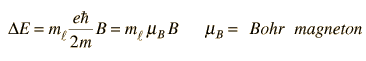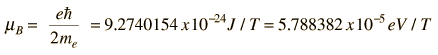# Why doesn't the normal Zeeman effect produce a band?

• B
I know that orbital angular momentum is quantized. If the potential energy is developed because of the external magnetic field applying a torque on the magnetic dipole, then from the dot product of the dipole moment and magnetic field we have a cosine term, shouldn't this give rise to all the energies corresponding to the various orientations that the orbital can have w.r.t the external magnetic field?

I know that orbital angular momentum is quantized. If the potential energy is developed because of the external magnetic field applying a torque on the magnetic dipole, then from the dot product of the dipole moment and magnetic field we have a cosine term, shouldn't this give rise to all the energies corresponding to the various orientations that the orbital can have w.r.t the external magnetic field?

in normal zeeman effect the orbital angular momentum states for a particular n (principal quantum number) -which has possibility of description in states of L=0,1,2,....(n-1) which are not split normally ,the external magnetic fields lifts the degeneracy in a manner that only those m(L) values are are available /allowed with m(L) taking -L, (L-1) ....0 ,1,2 ... +L in steps of 1 only.
these are the allowed states quantum mechanically
therefore the expectation that all cos(theta) values are possible is not allowed.

For example for L=1 a p state only m(L) are -1, 0, +1 making definite theta with the z axis (magnetic field axis) in a vector model.

thereby
Considering the quantization of angular momentum , this gives equally spaced energy levels displaced from the zero field level byso the spectrum can not be continuous and will be spaced by the above.
see; http://hyperphysics.phy-astr.gsu.edu/hbase/quantum/zeeman.html for an introductory treatment